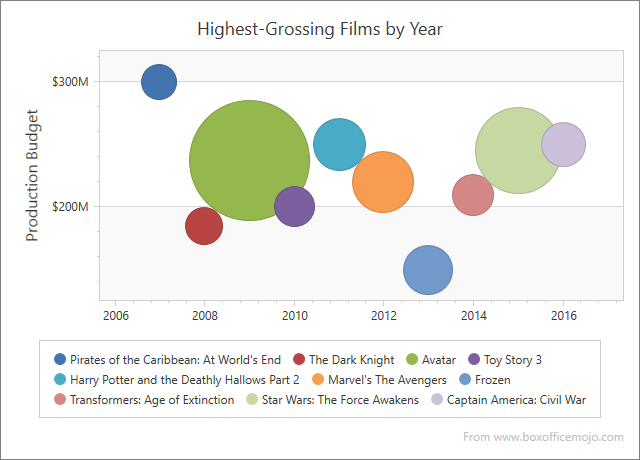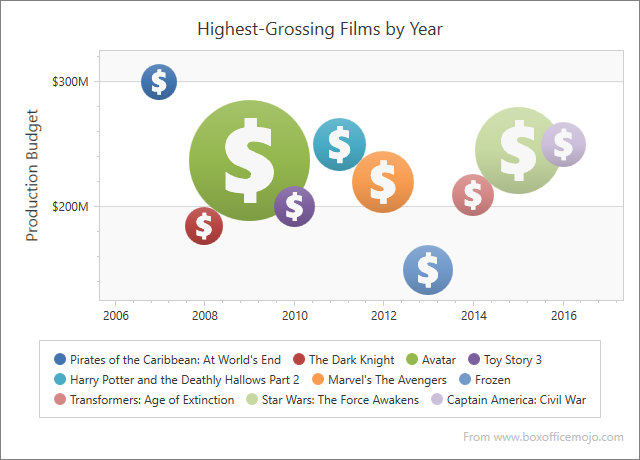# Bubble

## Description

The Bubble series allows you to display data with three dimensions: the position of each series point on the X and Y-axes indicates values of two dimensions, and the bubble’s size the third dimension.

The image below shows an example of a Bubble chart:Main characteristics:

Feature Value
Series View type BubbleSeries2D
Diagram type XYDiagram2D
Number of arguments per series point 1
Number of values per series point 2

## Create Bubble Series in Markup

The following example creates a 2D Bubble chart. Assign an XYDiagram2D object to the ChartControl.Diagram property and add a BubbleSeries2D object with points to the Diagram.Series collection.

This example also customizes the Y-axis’s whole range and a crosshair cursor.

``````<Window x:Class="Bubble2DChart.Window1" xmlns="http://schemas.microsoft.com/winfx/2006/xaml/presentation"
xmlns:x="http://schemas.microsoft.com/winfx/2006/xaml"
xmlns:dxc="http://schemas.devexpress.com/winfx/2008/xaml/charts" Title="Window1">
<Grid>
<dxc:ChartControl>
<dxc:ChartControl.CrosshairOptions>
<dxc:CrosshairOptions ShowValueLine="True" ShowValueLabels="True"/>
</dxc:ChartControl.CrosshairOptions>
<dxc:ChartControl.Diagram>
<dxc:XYDiagram2D>
<dxc:XYDiagram2D.Series>
<dxc:BubbleSeries2D Transparency="0.2"
AutoSize="False"
MinSize="1"
MaxSize="3"
ColorEach="True"
CrosshairLabelPattern="Argument: {A}; Value: {V}; Weight: {W}">
<dxc:BubbleSeries2D.Points>
<dxc:SeriesPoint Argument="A" Value="100" dxc:BubbleSeries2D.Weight="0.9" />
<dxc:SeriesPoint Argument="B" Value="60" dxc:BubbleSeries2D.Weight="0.8" />
<dxc:SeriesPoint Argument="C" Value="30" dxc:BubbleSeries2D.Weight="0.5" />
<dxc:SeriesPoint Argument="D" Value="43" dxc:BubbleSeries2D.Weight="0.4" />
<dxc:SeriesPoint Argument="E" Value="11" dxc:BubbleSeries2D.Weight="0.4"/>
<dxc:SeriesPoint Argument="F" Value="29" dxc:BubbleSeries2D.Weight="0.2"/>
<dxc:SeriesPoint Argument="G" Value="12" dxc:BubbleSeries2D.Weight="0.2"/>
<dxc:SeriesPoint Argument="H" Value="21" dxc:BubbleSeries2D.Weight="0.2"/>
<dxc:SeriesPoint Argument="I" Value="28" dxc:BubbleSeries2D.Weight="0.2"/>
</dxc:BubbleSeries2D.Points>
</dxc:BubbleSeries2D>
</dxc:XYDiagram2D.Series>
<dxc:XYDiagram2D.AxisY>
<dxc:AxisY2D GridLinesMinorVisible="True" >
<dxc:AxisY2D.WholeRange>
<dxc:Range MinValue="0" MaxValue="130"
SideMarginsValue="0" />
</dxc:AxisY2D.WholeRange>
</dxc:AxisY2D>
</dxc:XYDiagram2D.AxisY>
</dxc:XYDiagram2D>
</dxc:ChartControl.Diagram>
</dxc:ChartControl>
</Grid>
</Window>
``````

## Create Bubble Series in Code

Follow the steps below to create a 2D Bubble chart in code:

``````private void Window_Loaded(object sender, RoutedEventArgs e) {
// Create a chart.
ChartControl chart = new ChartControl();

// Create a diagram.
XYDiagram2D diagram = new XYDiagram2D();
chart.Diagram = diagram;

// Create a bubble series.
BubbleSeries2D series = new BubbleSeries2D();

// Add points to the series.
SeriesPoint point1 = new SeriesPoint("A", 70);
BubbleSeries2D.SetWeight(point1, 1);

SeriesPoint point2 = new SeriesPoint("B", 30);
BubbleSeries2D.SetWeight(point2, 1.7);

SeriesPoint point3 = new SeriesPoint("C", 60);
BubbleSeries2D.SetWeight(point3, 1.2);

SeriesPoint point4 = new SeriesPoint("D", 43);
BubbleSeries2D.SetWeight(point4, 0.8);

series.ColorEach = true;
series.Transparency = 0.1;
series.MarkerModel = new PentagonMarker2DModel();

// Add the chart to the window.
this.Content = chart;
}
``````

## Bind Bubble Series to a Data Source

Use the following properties to bind a 2D Bubble chart to data:

``````<Window
xmlns="http://schemas.microsoft.com/winfx/2006/xaml/presentation"
xmlns:x="http://schemas.microsoft.com/winfx/2006/xaml"
xmlns:d="http://schemas.microsoft.com/expression/blend/2008"
xmlns:mc="http://schemas.openxmlformats.org/markup-compatibility/2006"
xmlns:local="clr-namespace:BubbleChartDataBinding"
xmlns:dxc="http://schemas.devexpress.com/winfx/2008/xaml/charts" x:Class="BubbleChartDataBinding.MainWindow"
mc:Ignorable="d"
Title="MainWindow" Height="450" Width="800">
<Window.DataContext>
<local:ChartViewModel/>
</Window.DataContext>
<Grid>
<dxc:ChartControl>
<dxc:ChartControl.CrosshairOptions>
<dxc:CrosshairOptions ShowValueLine="True" ShowValueLabels="True"/>
</dxc:ChartControl.CrosshairOptions>
<dxc:ChartControl.Diagram>
<dxc:XYDiagram2D>
<dxc:XYDiagram2D.Series>
<dxc:BubbleSeries2D Transparency="0.2"
AutoSize="False"
MinSize="0.8"
MaxSize="2.7"
ColorEach="True"
DataSource="{Binding Data}"
ArgumentDataMember="Year"
ValueDataMember="Budget"
WeightDataMember="Grosses">
</dxc:BubbleSeries2D>
</dxc:XYDiagram2D.Series>
</dxc:XYDiagram2D>
</dxc:ChartControl.Diagram>
</dxc:ChartControl>
</Grid>
</Window>
``````
``````using System.Data;
using System.Windows;

namespace BubbleChartDataBinding {
public partial class MainWindow : Window {
public MainWindow() {
InitializeComponent();
}
}
public class ChartViewModel {
public DataTable Data {
get { return GetData(); }
}
public DataTable GetData() {
DataTable table = new DataTable();
table.Columns.AddRange(new DataColumn[] { new DataColumn("Year", typeof(int)),
new DataColumn("Budget", typeof(decimal)),
new DataColumn("Grosses", typeof(decimal)),
new DataColumn("Title", typeof(string)) });

table.Rows.Add(2007, 300, 0.963, "Pirates of the Caribbean: At World's End");
table.Rows.Add(2008, 185, 1.004, "The Dark Knight");
table.Rows.Add(2010, 200, 1.067, "Toy Story 3");
table.Rows.Add(2011, 250, 1.341, "Harry Potter and the Deathly Hallows Part 2");
table.Rows.Add(2012, 220, 1.519, "Marvel's The Avengers");
table.Rows.Add(2014, 210, 1.104, "Transformers: Age of Extinction");
table.Rows.Add(2015, 245, 2.068, "Star Wars: The Force Awakens");
table.Rows.Add(2016, 250, 1.153, "Captain America: Civil War");
return table;
}
}
}
``````

## Configure Bubble Size

The Chart Control calculates a bubble’s size based on the Weight value. Set the BubbleSeries2D.AutoSize property to false to specify maximum and minimum bubble sizes. To do this, use the BubbleSeries2D.MaxSize and BubbleSeries2D.MinSize properties. The maximum and minimum values are calculated in X-axis units.

``````<dxc:BubbleSeries2D AutoSize="False" MinSize="1" MaxSize="3">
<!--...-->
</dxc:BubbleSeries2D>
``````

## Specify Point Marker Type

You can use different shapes (crosses, stars, triangles, etc.) to display series points. To do this, specify the MarkerModel property:

``````<dxc:BubbleSeries2D>
<dxc:BubbleSeries2D.MarkerModel>
<dxc:DollarMarker2DModel/>
</dxc:BubbleSeries2D.MarkerModel>
</dxc:BubbleSeries2D>
``````

Result:All available models are listed in the following help topic: Series and Marker Models: Predefined Models for Series Markers.

You can also specify a custom model as described in the following section: Series and Marker Models: Custom Model for 2D Series Markers.

Note

For information on which series view types can be combined with the Bubble view, refer to the following document: Series Type Compatibility.